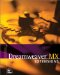# Variables and Expressions

### Variables and Expressions

A variable is a container that holds a piece of data (called the value ). An expression is the part of a JavaScript statement that evaluates the variable, substituting the value for the variable where necessary. In JavaScript, we use the keyword var to create a variable, and the equal sign ( = ) to assign a value to a variable. Here is a typical group of statements using variables:

` var x = 6;  var y = 3;  var z = x + y;  x = x + 1;  y = y + 1;  var a = x + y; `

At the end of this group of statements, the variable z is equal to (contains) 9; a is equal to 11.

A variable can contain different types of information, including numbers , pieces of text called strings , and true/false values called Booleans . Depending on the kind of information contained in a variable, the JavaScript interpreter evaluates the expression containing it differently. When variables contain numbers, for instance, as in the preceding example, the + operator means to perform a mathematical addition operation. When variables contain text strings, the + operator means to join the two strings together, as follows :

` var a = "inter";  var b = "mediate";  var c = a + b; `

At the end of this group of statements, the variable c is equal to the text string "intermediate." This process of joining text strings is called concatenation . Variables can be concatenated with each other and with other text strings, like this:

` var d = "Fred";  var e = "Barney";  var f = d + " and " + e; `

At the end of this group of statements, the variable f is equal to the text string " Fred and Barney ". The word and , as well as the spaces on either side of this word, are contained in the text string that is joined to, or concatenated with, the two variables.

#### Practice Session #2

In this exercise, you'll build on the practice file you created in the previous exercise, using variables to construct the message that appears in the alert window.

1. In your text editor, open practice1.htm and save it as practice2.htm.

2. Change the contents of the <script> tag to look like this (new code is in bold):

` <script language="JavaScript">  var person = "Fred";   var greeting = "Hello, " + person + "!";   alert(greeting);  </script> `
3. Save the file and try it out in a browser. The resulting alert window should look like the one shown in Figure A.2. Can you see how the extra space at the end of the Hello, text string creates the space between the words in the final message?

##### Figure A.2. The alert window for practice2.htm, concatenating variables to create the alert message.Dreamweaver MX Extensions
ISBN: 0735711828
EAN: 2147483647
Year: 2001
Pages: 141
Authors: Laura Gutman

Similar book on Amazon

flylib.com © 2008-2017.
If you may any questions please contact us: flylib@qtcs.net